Get instant live expert help with Excel or Google Sheets“My Excelchat expert helped me in less than 20 minutes, saving me what would have been 5 hours of work!”

#### Post your problem and you’ll get Expert help in seconds.

Your message must be at least 40 characters
Our professional Expert are available now. Your privacy is guaranteed.

# Rank with Ordinal Suffix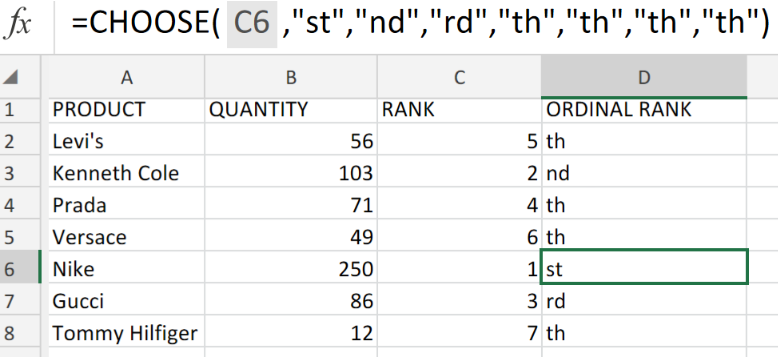Figure 1. of Rank with Ordinal Suffix.

## Generic Formula

This Excel Function is two-fold!

1. If we are required to list certain items according to their rank (least to largest) in Excel, we are going to utilize the RANK Function.

`=RANK(number,range)`

1. If we are required to list certain items in ordinal manner (1st, 2nd, 3rd, etc,) with suffixes in Excel, we are going to utilize the CHOOSE Function.

`=CHOOSE(number,range)`

## How to use the RANK and CHOOSE Functions in Excel.

Our purpose here is to provide Ranking with Ordinal Suffix for a number of clothing items in a shop. We are going to utilize both the RANK and CHOOSE Functions in our worksheet to achieve our desired results.

We can do this by following five simple steps.

1. Collect the data values available for ranking and arrange within the columns of our worksheet. See example illustrated below;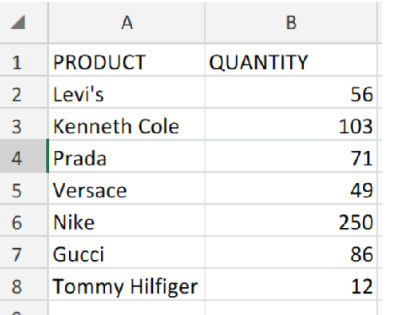Figure 2. of Clothing Items for Ranking in Excel.

1. Label the next column, RANK, where Excel will determine the ranking of the clothing items according to their quantity. The RANK formula which we will enter into the formula bar for cell C2 of our worksheet is as follows; =RANK(B2,B2:B8)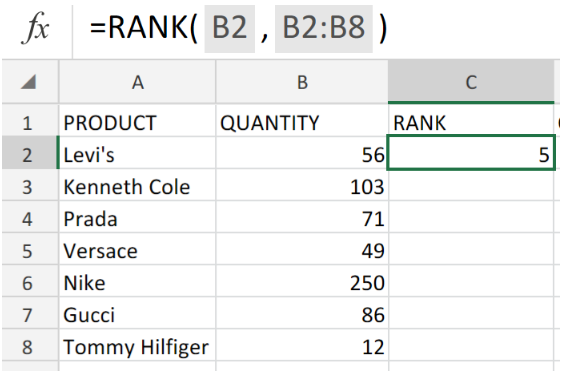Figure 3. of RANK Function in Excel.

1. Modify and copy the RANK formula in cell C2 into the other cells in the RANK column to achieve our desired results.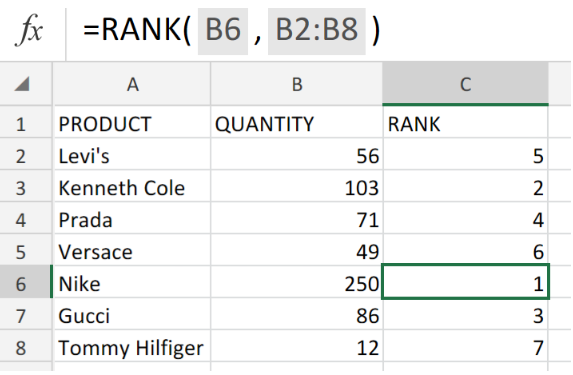Figure 4. of RANK Function in Excel.

1. Label another column, ORDINAL RANK, where Excel can list the items in ordinal manner and attach the appropriate suffixes. The CHOOSE formula we are going to enter into the formula bar for cell D2 of our worksheet, is as follows;

`=CHOOSE(C2,"st","nd","rd","th","th","th","th")`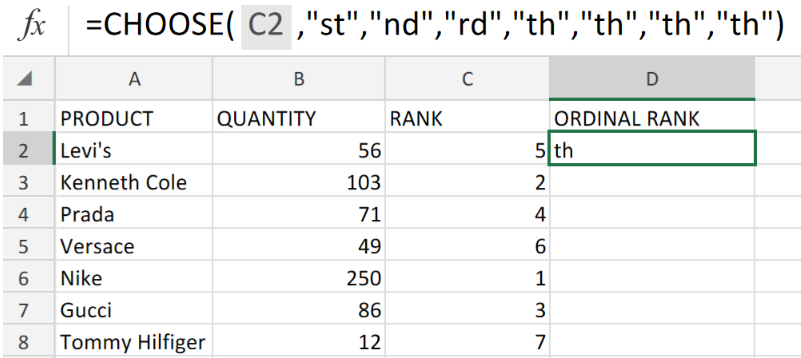Figure 5. of CHOOSE Function in Excel.

1. Modify and copy the CHOOSE formula in cell D2, into the other cells in the ORDINAL RANK column to get the desired results.Figure 6. of RANK and CHOOSE Functions in Excel.

Ordinal number values signify a sequential order of rank or position. Commonly written as a number followed by a letter suffix (1st, 2nd, 3rd, etc).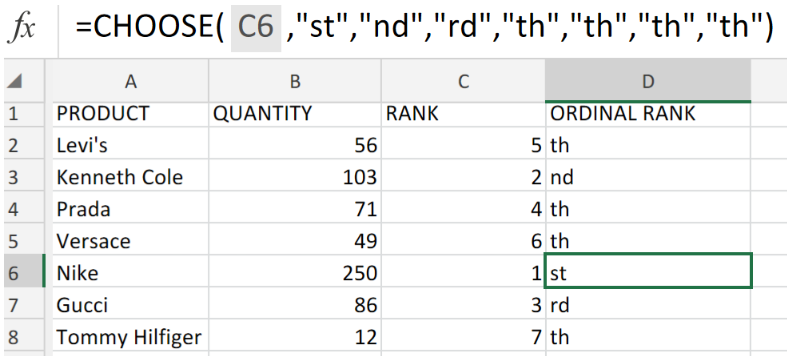Figure 7. of Final Result.

## Instant Connection to an Expert through our Excelchat Service

Our live Excelchat Service is here for you. We have Excel Experts available 24/7 to answer any Excel questions you may have. Guaranteed connection within 30 seconds and a customized solution for you within 20 minutes.

### Did this post not answer your question? Get a solution from connecting with the expert.Another blog reader asked this question today on Excelchat:
Solution examplesim creating a fantasy hockey model and trying to figure out how to rank if someone has played more than a certain number of games, and if they're certain position how to rank them accordingly
Solved by X. D. in 60 minsI cannot enter this formula into Excel. I get an error message asking for an apostrophe?IF(\$S2=0,"",IF(\$S2>0,RANK(\$S2,\$S\$2:\$S\$8000),RANK(\$S2,\$S\$2:\$S\$8000-COUNTIF(\$S\$2:\$S\$8000,0)))
Solved by B. W. in 20 mins## Subscribe to Excelchat.coAnother blog reader asked this question today on Excelchat: